Command Reference : Matrix Language Reference

 @ones
Matrix of vector of ones.
Syntax: @ones(n1, n2)
n1: integer
n2: integer
Return: matrix, vector
Creates a matrix or vector filled with the value 1. The size of the created matrix is given by the integers n1 (number of rows) and n2 (number of columns).
Examples
matrix m1 = @ones(3,2)
creates M1, a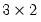matrix of ones.
sym s1 = @ones(5, 5)
sym s2 = @unvech(@ones(5*6/2))
create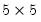symmetric matrices of ones, while
vector v1 = @ones(18)
is an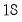element vector ones, and
matrix k4 = @kronecker(@fill(1, 2, 3), @ones(4, 4))
generates the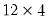matrix formed by taking the Kronecker product of the 3 element vector and the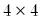matrix of ones.
Cross-references